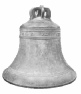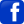﻿ Sound of Bells – Formulae governing soundUK Registered Charity Number: 1154107Conservation by Re-use

Helping churches acquire surplus and/or redundant bells to be hung for

English-style full-circle bell-ringing.Index Previous page Next page

Sound of Bells – Formulae governing sound

The frequency, intensity and rate of decay of each partial depends on the mouth diameter, height, shape, thickness profile, metal composition and casting imperfections. The general theorem governing the sound of a bell7 is :-

f = k1t      (     E       1     )                                Equation 1

d2          ( s   (1-m2)     )

where :

f = frequency

kx = constants (different)

t = thickness of bell at soundbow

d = diameter of bell (at the mouth)

E = Youngs modulus (stiffness factor for material)

s = Density (inertia factor of material)

m = Poisson's ratio (lateral contraction per unit breadth divided by longitudinal elongation per unit length)

Equation 1 shows the frequency is proportional to the thickness and inversely proportional to the square of the diameter. The weight of a bell is dependent on the height, diameter, thickness profile and the density of the metal. Bell-founders usually cast bells where the height and thickness are directly related to the diameter. For example the soundbow thickness might be one thirteenth of the diameter and the height might be 5/6ths of the diameter. If "t" in equation 1 is replaced by "d/13" then the equation simplifies to :

f = k2      (  E       1    )                                      Equation 2

d          ( s   (1-m2) )

For a series of bells which use the same metal composition and where the diameter, thickness and height change in equal proportions then the weight will vary with the cube of the diameter. Two bells in such a series can be compared by the following equation :-

f1 = d2 f2                                       Equation 3

d1

where :

fx = frequency of bell "x"

dx = diameter of bell "x"

Bells cast to this scale will have the frequency inversely proportional to the diameter. For two bells an octave apart, the diameter of the larger bell would be twice that of the smaller bell and the weight would be eight times that of the smaller bell. This scale is often used for all except the lighter bells of a Carillon. If this scale were used for ringing bells then for a tenor of weight 12cwt, the treble to the octave would weigh only 1.5cwt. This scale is unsuitable for ringing bells because of the disproportionate handling of the trebles compared to the tenors and more importantly, the insufficient sound strength from the trebles.